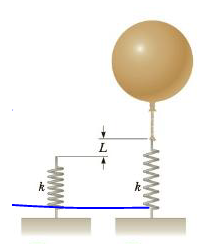Chapter 9, Problem 87AP

Chapter
Section
Textbook Problem

A light spring of constant A = 90.0 N/m is attached vertically to a table (Fig. P9.87a). A 2.00-g balloon is filled with helium (density = 0.179 kg/m3) to a volume of 5 00 m3 and is then connected to the spring, causing the spring to stretch as shown in Figure P9.87b. Determine the extension distance L when the balloon is in equilibrium.To determine
The extension distance L when the balloon is in equilibrium.

Explanation
At equilibrium state of the balloon, the sum of forces on the balloon is BFsFg,balloonFg,He=0 . Let us give the expression for each force on the balloon by starting from the upward force of buoyancy B=(ρairVballoon)g and all three downward forces are Fg,balloon=mg , Fg,He=(ρHeVballoon)g , and the spring force Fs=k|Δx|=kL . Using all these expressions, the extension distance of the spring can be written as L=[(ρairρHe)Vballoonm]g/k .

Given info: The constant of spring is 90.0N/m , mass of the balloon is 2.00kg , density of helium is 0.179kg/m3 , density of air is 1.29kg/m3 , volume of the balloon is 5.00m3 , and acceleration due to gravity is 9.80m/s2 .

The formula for the extension distance of the spring when the balloon in equilibrium is,

L=[(ρairρHe)Vballoonm]gk

• ρair is density of air

Still sussing out bartleby?

Check out a sample textbook solution.

See a sample solution

The Solution to Your Study Problems

Bartleby provides explanations to thousands of textbook problems written by our experts, many with advanced degrees!

Get Started